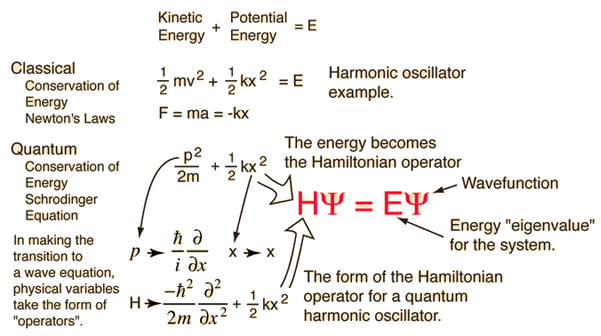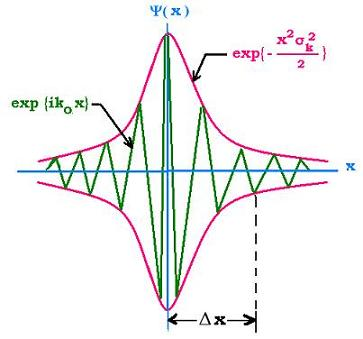# This note has been used to help create the Schrödinger Equation wikiFrom Wave to Particle

Louis de Broglie taught us that all particles in the universe possess a wavelength. We will consider microscopic objects because they closely follow the wave-particle duality more than macroscopic objects. Consider a particle travelling in space with no potential holding it down: we will call this the free particle. Since microscopic particles also behave as a wave, we will model the free particle as a plane wave enveloped in a corresponding wave-packet. The plane wave propagates in space and evolves in time: $\Psi (x,t) = {e}^{i(kx-\omega t)}$ with a corresponding wave-packet: $\Psi(x,t) = \frac{1}{\sqrt{2\pi}}\int_{-\infty}^{\infty}{\phi(k)}{e}^{i(kx-\omega t) }dk.$Wave packet

Using de Broglie’s equations of momentum $p=\hbar k=\frac{h}{\nu}$ and energy $E= \hbar \omega = \frac{{p}^{2}}{2m}$ we can rewrite the wave-packet equation as $\Psi(x,t) = \frac{1}{\sqrt{2\pi \hbar}}\int_{-\infty}^{\infty}{\phi(p)}{e}^{i(px-Et)/ \hbar}dp.$

What can we do with this integral so that we can describe a physical system? Why not take derivatives until two sides of an equation match? Let’s begin by taking the time derivative and two derivatives in space: $\frac { \partial \Psi }{ \partial t } =\frac { 1 }{ \sqrt { 2\pi \hbar } } \int _{ -\infty }^{ \infty }{ \phi (p){ \left( \frac { -iE }{ \hbar } \right) e }^{ i(px-Et)/\hbar }dp }$

$\frac { \partial \Psi }{ \partial x } =\frac { 1 }{ \sqrt { 2\pi \hbar } } \int _{ -\infty }^{ \infty }{ \phi (p){ \left( \frac { ip }{ \hbar } \right) e }^{ i(px-Et)/\hbar }dp }$

$\frac { { \partial }^{ 2 }\Psi }{ \partial { x }^{ 2 } } =\frac { 1 }{ \sqrt { 2\pi \hbar } } \int _{ -\infty }^{ \infty }{ \phi (p){ \left( \frac { -{ p }^{ 2 } }{ { \hbar }^{ 2 } } \right) e }^{ i(px-Et)/\hbar }dp }.$

Now there is one final step to do: balancing. If we multiply the time derivative by $i\hbar$ and the second spatial derivative by $\frac{-{\hbar}^{2}}{2m}$, we get

$i\hbar \frac { \partial \Psi }{ \partial t } = \frac{-{\hbar}^{2}}{2m}\frac { { \partial }^{ 2 }\Psi }{ \partial { x }^{ 2 } }$

(this can be easily verified using the de Broglie equations).

It is customary in physics to describe physical systems in terms of total energy, which is the sum of the kinetic and potential energies. So all we have to do to this free particle is add $V\Psi$ to the right hand side. Hence,

$i\hbar \frac { \partial \Psi }{ \partial t } = \frac{-{\hbar}^{2}}{2m}\frac { { \partial }^{ 2 }\Psi }{ \partial { x }^{ 2 } }+ V\Psi.$

Operators

Quantum mechanics is inherently linear, which means linear algebra (more specifically linear operator algebra) is the language of QM. Thus, it is most appropriate to write the Schrödinger equation in operator form.

An operator is an instruction that is acted on a function. An operator $\hat{A}$ is linear if it satisfies the identity $\hat{A}[af(x)+bg(x)] = a\hat{A}f(x)+b\hat{A}g(x)$where $a,b$ are scalars. This is where QM starts to get abstract, so I will introduce some concrete examples. Consider the operators $\hat{p}=-i\hbar \frac{\partial}{\partial x}$ and $\hat{x}=x$ If we act $\hat{p}$ on some position quantity $x$, we get the differential $-i\hbar \frac{\partial x}{\partial x} = -i\hbar.$ Hence, momentum is a differential operator. Looks straightforward enough! But consider what we can do to operators ${\hat{p}}^{2} = {\left(-i\hbar \frac{\partial}{\partial x}\right)}^{2}$

${\hat{p}}^{2}= -{\hbar}^{2} \frac{{\partial}^{2}}{\partial {x}^{2}}$ which is convenient. Now let us look at the Schrödinger equation again…in operator form

$i\hbar \frac { \partial \Psi }{ \partial t } =\left[\frac{{\hat{p}}^{2}}{2m}+ \hat{V} \right] \Psi .$

For the time-independent case , we replace the time derivative term with total energy, and if we want to be even more compact, we may denote the operator on the right hand side with the Hamiltonian operator $\hat{H} = \hat{T} +\hat{V}$.

$E\Psi=\hat{H} \Psi .$

Notice how this is shorter? Well, that's good and all, but not the point. The point is that we are acting a Hamiltonian operator on the wavefunction $\Psi$, which is convenient for reasons unclear at the moment. You see, linear operators can describe many things, but all linear operators follow the same algebra. We have entered the realm where abstract algebra infiltrates all of physics for the convenience of problem solving. So from now on, we consider operators to act on physical quantities and objects.

Determinate States

When you solve the time-independent Schrödinger equation, you will encounter values of energy that satisfy $E\Psi=\hat{H} \Psi.$

These are called determinate states, which are eigenfunctions (also called eigenstates) of the linear operator $\hat{H}$ with corresponding energy eigenvalues $E$. When a linear operator possess several eigenvalues, we call the set of eigenvalues its spectrum. If two or more linearly independent eigenfunctions share the same eigenvalue, we call those spectra degenerate. You will later learn that degeneracies play an important role in describing the atom; hence much of quantum chemistry and solid-state physics. But for starters, you should solve the 1D particle in a box (Problem 3) below and calculate the corresponding energy eigenvalues ${E}_{n}$.Visit my set Lectures on Quantum Mechanics for more notes.

Problems

1. Use the de Broglie relations to obtain the dispersion relation of wave-packets. Hint: $\omega \propto {k}^{2}$

2. 1D Particle in a Box Consider the potential that is 0 within the region $0 and infinite elsewhere. Solve the time-independent Schrödinger equation for this potential with boundary conditions: $\Psi(0,t) = \Psi(a,t) = 0$ . Once you find the wavefunction (solution), normalize the wavefunction $\Psi(x,0)$.

Note: $\Psi(x,t)$ is just $\Psi(x,0) {e}^{-iE t/\hbar}$

3. Now that you found the solution to the 1D Particle in a Box, find the set permissible eigenenergies (energy levels). Remember that the wavenumber of a matter-wave is given by $k=\frac{n\pi}{a}$ where $n=1,2,3,...$. This will help you simplify ${E}_{n}$.Note by Steven Zheng
5 years, 10 months ago

This discussion board is a place to discuss our Daily Challenges and the math and science related to those challenges. Explanations are more than just a solution — they should explain the steps and thinking strategies that you used to obtain the solution. Comments should further the discussion of math and science.

When posting on Brilliant:

• Use the emojis to react to an explanation, whether you're congratulating a job well done , or just really confused .
• Ask specific questions about the challenge or the steps in somebody's explanation. Well-posed questions can add a lot to the discussion, but posting "I don't understand!" doesn't help anyone.
• Try to contribute something new to the discussion, whether it is an extension, generalization or other idea related to the challenge.

MarkdownAppears as
*italics* or _italics_ italics
**bold** or __bold__ bold
- bulleted- list
• bulleted
• list
1. numbered2. list
1. numbered
2. list
Note: you must add a full line of space before and after lists for them to show up correctly
paragraph 1paragraph 2

paragraph 1

paragraph 2

[example link](https://brilliant.org)example link
> This is a quote
This is a quote
    # I indented these lines
# 4 spaces, and now they show
# up as a code block.

print "hello world"
# I indented these lines
# 4 spaces, and now they show
# up as a code block.

print "hello world"
MathAppears as
Remember to wrap math in $$ ... $$ or $ ... $ to ensure proper formatting.
2 \times 3 $2 \times 3$
2^{34} $2^{34}$
a_{i-1} $a_{i-1}$
\frac{2}{3} $\frac{2}{3}$
\sqrt{2} $\sqrt{2}$
\sum_{i=1}^3 $\sum_{i=1}^3$
\sin \theta $\sin \theta$
\boxed{123} $\boxed{123}$

Sort by:

Nice presentation. It'd be great if you could show how Schrodinger's equation is the same as the diffusion equation in classical mechanics, but in complex time. This is the reason why Richard Feynman thought that quantum behavior could be modeled as a random walk in complex space. Too bad more isn't being said about this.

- 5 years, 10 months ago

I think the reason why more hasn't been said on Feynman's idea is that the probabilistic interpretation of QM showed infallible accuracy. The diffusion equation still relates in the probabilistic interpretation, since the wave-packet of observed quantities disperse if you stop observing for a while.

- 5 years, 10 months ago

What Feynman actually said about attempting to model it as a random walk in complex space, you first need a quantum computer---and back in those days, "quantum computers" was just a wildly speculative idea. His point was that you can't duplicate quantum behavior using classical means. But we need to distinguish "duplicate quantum behavior" from making predictions as per equations of quantum mechanics, the latter quite doable with conventional computers.

- 5 years, 10 months ago

Is there any pdf or a source or website where these algebra are explained from scratch, i am familiar with grad, div, curl and greens and stokes theorem and their elementary applications in finding line and surface integrals, so anything to build upon that ?

thank you,

- 5 years, 10 months ago

div,grad, curl, laplacians are differential operators but operator algebra is much more general and diverse. There are too many operator algebras. IN QM, we focus on linear operator algebra.

- 5 years, 10 months ago

@Steven Zheng Nice lectures Could you please upload a few more problems on QM.

- 5 years, 6 months ago